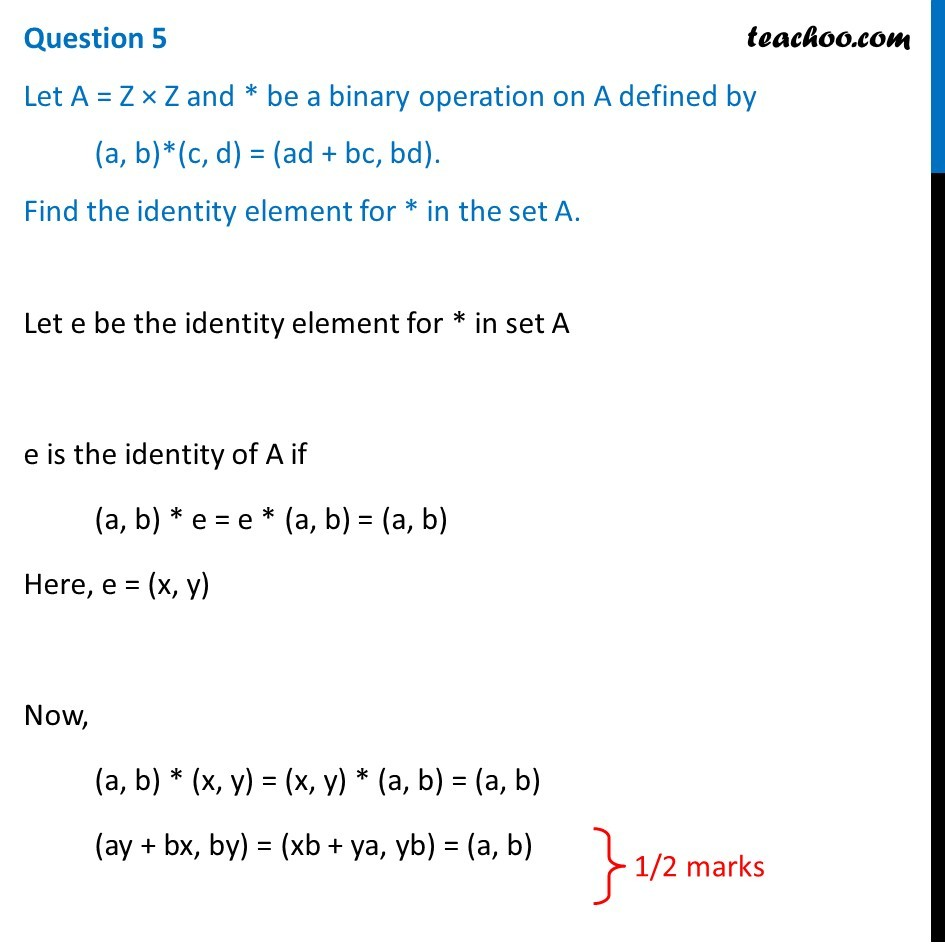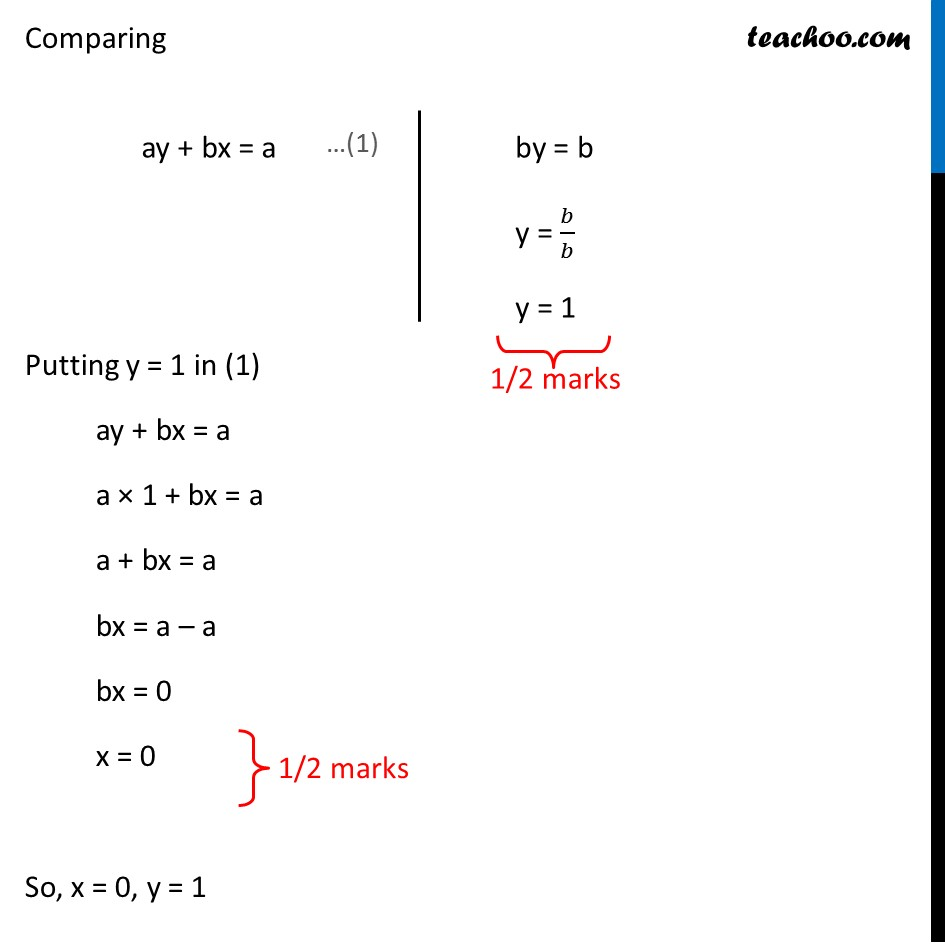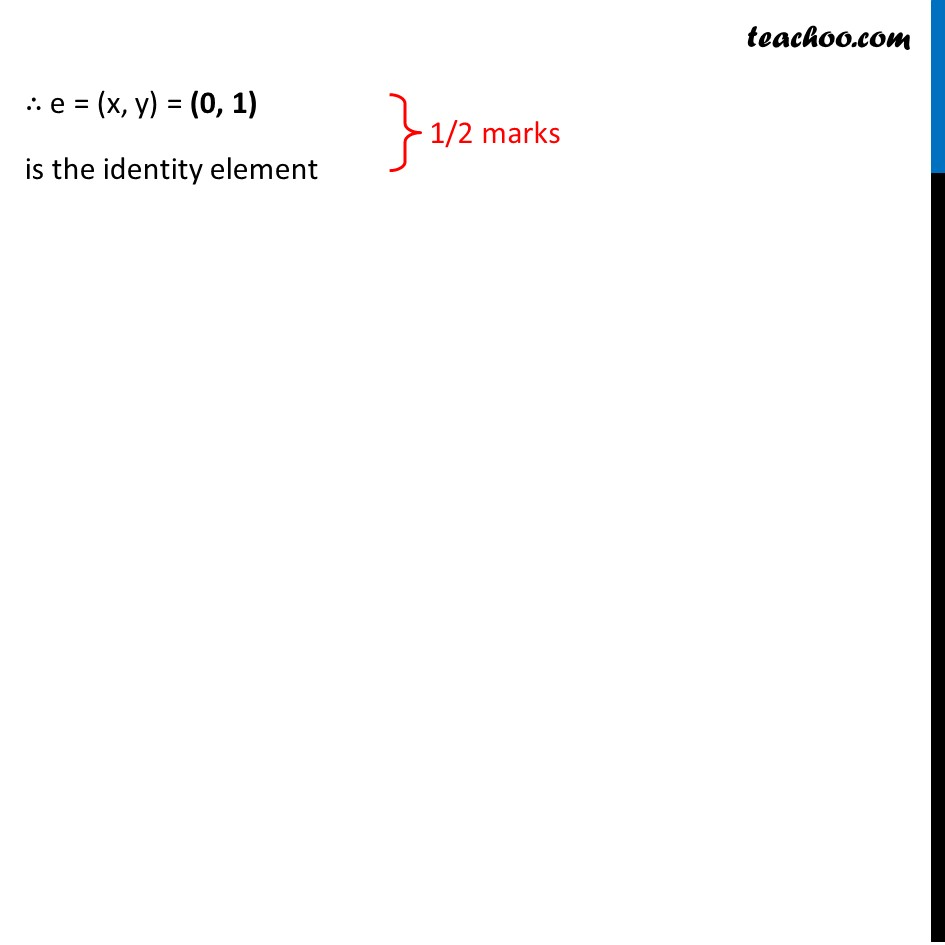CBSE Class 12 Sample Paper for 2019 Boards

Class 12
Solutions of Sample Papers and Past Year Papers - for Class 12 Boards

Question 5

Let A = Z × Z and * be a binary operation on A defined by

(a, b)*(c, d) = (ad + bc, bd).

Find the identity element for * in the set A.Get live Maths 1-on-1 Classs - Class 6 to 12

### Transcript

Question 5 Let A = Z × Z and * be a binary operation on A defined by (a, b)*(c, d) = (ad + bc, bd). Find the identity element for * in the set A. Let e be the identity element for * in set A e is the identity of A if (a, b) * e = e * (a, b) = (a, b) Here, e = (x, y) Now, (a, b) * (x, y) = (x, y) * (a, b) = (a, b) (ay + bx, by) = (xb + ya, yb) = (a, b) Comparing ay + bx = a by = b y = 𝑏/𝑏 y = 1 Comparing Putting y = 1 in (1) ay + bx = a a × 1 + bx = a a + bx = a bx = a – a bx = 0 x = 0 So, x = 0, y = 1 ∴ e = (x, y) = (0, 1) is the identity element